# Chapter 5 - Magnetism and Matter

Magnetic forces exist throughout the universe, affecting everything from distant galaxies to tiny atoms. The Earth’s magnetic field existed before the evolution of humans. The term “magnet” originated from the Greek island of Magnesia, where magnetic ore was discovered as early as 600 BC. On this island, shepherds reported their wooden shoes with nails, and iron-tipped rods, getting stuck to the ground due to the attractive force of magnets, making it challenging for them to move.

The directional ability of magnets has been known since ancient times. When a thin, long magnet was suspended freely, it pointed in the north-south direction, and the same was observed when it was placed on a piece of cork floating in still water. Chinese texts dating back to 400 BC mention using magnetic needles for navigation by ships and caravans in the Gobi desert. The name “lodestone,” meaning leading stone, was given to a naturally occurring iron-magnetite ore. The Chinese are credited with exploiting this property technologically.

Magnetism is a force created by magnets due to their attraction or repulsion caused by the movement of electric charges. All substances consist of tiny units called atoms, which have electrons carrying electric charges. The electrons spin around the nucleus of an atom, creating an electric current and making each electron behave like a tiny magnet.

Materials like cloth or paper have weak magnetism due to equal numbers of electrons spinning in opposite directions, cancelling each other out. However, substances like iron, cobalt, and nickel have most electrons spinning in the same direction, resulting in strong atom magnetism but not yet magnetism. To become magnetised, they must be in the magnetic field of a strong magnet, which is the area around the magnet with magnetic force.

Important Questions For Class 12 Physics Chapter 5 Magnetism and Matter are provided here. Students must review and solve these questions to prepare for their Physics papers. They can also refer to these questions for quick revision. These questions are more likely to be asked in the exam; hence, students must practise them thoroughly.

## Very Short Answer Type Questions

1. The Earth acts as a magnet, with its magnetic field roughly directed from its geographic position

(a) North to South

(b) South to North

(c) East to West

(d) West to East

2. The intensity of the magnetic field of the Earth is

(a) constant everywhere.

(b) zero everywhere.

(c) having very high value.

(d) vary from place to place on the surface of the Earth.

Answer: (d) vary from place to place on the surface of the Earth.

3. What is the cause of the Earth’s magnetic field?

(a) Diversive current in the core of the Earth.

(b) Convective currents in the core of the Earth.

(c) Translational motion of the Earth.

(d) Rotational movement of the Earth.

Answer: (b) Convective currents in the core of the Earth

4. Which of the following standalone values is not employed to describe the Earth’s magnetic field?

(a) Magnetic dip δ

(b) Magnetic declination θ

(c) The vertical component of the field of the Earth BV

(d) Horizontal component of the field of Earth BH

Answer: (c) Vertical component of the field of the Earth BV

5. Which of the following characteristics demonstrates the nature of ferromagnetic substances?

(a) Ferromagnetic behaviour varies with temperature.

(b) Ferromagnetic behaviour is independent of temperature.

(c) Ferromagnets transform into diamagnets at sufficiently high temperatures.

(d) Ferromagnets become paramagnets at low temperatures.

Answer: (a) Ferromagnetic behaviour varies with temperature.

6. What is accurate regarding magnetic monopoles?

(a) A magnetic monopole exists.

(b) A magnetic monopole does not exist.

(c) Magnetic monopoles possess a constant monopole momentum.

(d) The monopole momentum increases as its distance from the field increases.

Answer: (b) A magnetic monopole does not exist.

1. A proton has a spin and magnetic moment, just like an electron. Why, then, is its effect neglected in magnetism of materials?

Solution: Protons have a spin and magnetic moment, similar to electrons, but in most materials, electrons are the main contributor to magnetism as they are more mobile and can be affected by external magnetic fields more easily than protons. The protons are typically located in the nuclei of atoms, and their magnetic moments are fixed and not affected by external magnetic fields as much as electron magnetic moments. Therefore, protons are usually not taken into account in the magnetism of materials, and the electron magnetic moments are given more importance.

2. A permanent magnet in the shape of a thin cylinder of length 10 cm has M = 106A/m. Calculate the magnetisation current IM.

Solution: The formula required to solve this question is I M = M I

It is given that the length of the cylinder (l) is equal to 0.1m.

The magnetic moment of the given cylinder, M = 106 Am-1

The magnetic current, IM = M I = 10 6 × 0.1 = 105 A

3. Explain quantitatively the order of magnitude difference between the diamagnetic susceptibility of N2 (~ 5 × 10-9) (at STP) and Cu (~ 10-5).

Solution: The density of nitrogen is

$$\begin{array}{l} \rho _ { N _ { 2 } } = \frac{ 28\, g }{ 22.4\,litres } \end{array}$$

$$\begin{array}{l} = \frac{ 28 g }{ 22400 cc } \end{array}$$

It is known that the density of copper is

$$\begin{array}{l} \rho _ { c u } = 8g / cc \end{array}$$

$$\begin{array}{l} \textup{Therefore, } \frac{ \rho _ { N _ { 2 } } }{ \rho _ { Cu } } = \frac{ 28 }{ 22400 } \times \frac{ 1 }{ 8 } \end{array}$$

$$\begin{array}{l} = 1.6 \times 10 ^ {-4} \end{array}$$

It is given that,

$$\begin{array}{l} \frac{ \chi _ { N _ { 2 } } } { \chi _ { cu } } = \frac{ 5 \times 10 ^ { -9 } }{ 10 ^ { -5 } } \end{array}$$

$$\begin{array}{l} = 5 \times 10 ^ { -4 } \textup{ ….. (i)} \end{array}$$

It is also known that

$$\begin{array}{l} \chi = \frac{ \textup{intensity of magnetisation } (I) }{\textup{magnetising force }(H) } \end{array}$$

$$\begin{array}{l} = \frac{\textup{magnetic moment(M) / volume (V)} }{H} \end{array}$$

$$\begin{array}{l} = \frac{ M }{ H V } = \frac{ M }{ H [mass(m)) \, / \, density (\rho) ]} =\frac{ I }{ H } \end{array}$$

$$\begin{array}{l} = \frac{ M }{ H V } = \frac{ M }{ H [mass(m)) \, / \, density (\rho) ]} =\frac{ I }{ H } \end{array}$$

$$\begin{array}{l} = \frac{ M }{ H V } = \frac{ M }{ H [mass(m)) \, / \, density (\rho) ]} = \frac{M _ { \rho }}{ H m } \end{array}$$

$$\begin{array}{l} \textup{Therefore, } \chi \propto \rho \textup{for the given value of } M \, / \, H m \end{array}$$

$$\begin{array}{l} \frac{ \chi _ { N _ { 2 } } }{ \chi _ { Cu } } = \frac{ \rho _ { N _ { 2 } } }{ \rho _ { Cu } } \end{array}$$

$$\begin{array}{l} = 1.6 \times 10 ^ { -4 } \textup{ …..(ii)} \end{array}$$

From equations (i) and (ii), we can see that the major variation in the susceptibility of Cu and N2 is due to their density.

4. From a molecular viewpoint, discuss the temperature dependence of susceptibility for diamagnetism, paramagnetism and ferromagnetism.

Solution: Diamagnetism is the result of the orbital motion of electrons creating magnetic moments that are opposite to an applied field. Therefore, it is not greatly impacted by temperature changes.

The alignment of atomic magnetic moments in the direction of an applied field causes paramagnetism and ferromagnetism. However, as the temperature increases, this alignment is disrupted, leading to a decrease in the susceptibility of both types of magnetism with increasing temperature.

5. A ball of superconducting material is dipped in liquid nitrogen and placed near a bar magnet.

(i) In which direction will it move?

(ii) What will be the direction of its magnetic moment?

Solution: Superconducting materials and liquid nitrogen are both diamagnetic. When a superconducting ball is submerged in liquid nitrogen, it acts as a diamagnetic substance. When placed near a bar magnet, it will weakly be magnetised in the direction opposite to the magnetic field. As a result, it will move away from the magnet, and its magnetic moment will be in the opposite direction to the magnetic field of the magnet.

1. Verify Gauss’s law of the magnetic field of a point dipole of dipole moment at the origin for the surface, which is a sphere of radius R.

Solution: Gauss’s law of magnetism gives us that

$$\begin{array}{l} \oint \vec{ B } . d \vec{ S } = 0 \end{array}$$

Then, the magnetic dipole moment of the dipole situated at origin point “O” is along the z-axis

$$\begin{array}{l} \vec{ M } = M \hat{ k } \end{array}$$

Take P be as a point at length r from the point O and point OP due to dipole moment

$$\begin{array}{l} \vec{ M } \cos \theta \textup{ is } \vec{ B } = \frac{ \mu _ { 0 } }{ 4 \pi } \frac{ 2 M cos \theta }{ r ^ { 3 } } \hat{ r } \end{array}$$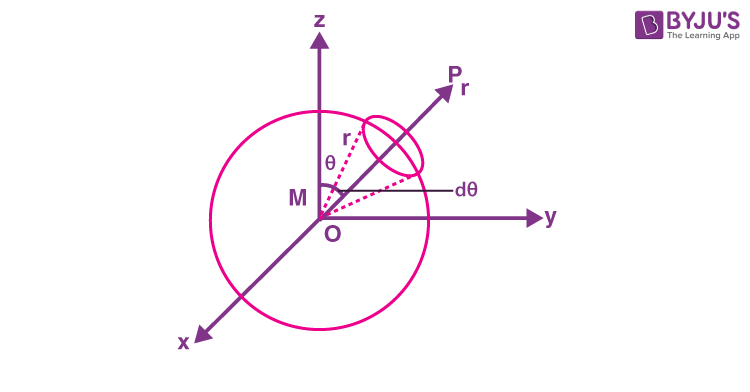From the figure, r is the sphere radius with the centre at point O existing in yz-plane. Consider an elementary area dS of the surface at point P, then

$$\begin{array}{l} d \vec{ S } = r ( r sin \theta ) \hat{ r } = r ^ { 2 } \sin \theta d \theta \hat{ r } \end{array}$$

$$\begin{array}{l} \textup{Therefore, } \oint \vec{ B } . d \vec{ S } = \oint \frac{ \mu _ { 0 } }{ 4 \pi } \frac{ 2 M \cos \theta }{ r ^ { 3 } } \hat{ r } \, ( r ^ { 2 } \sin \theta d \theta ) \end{array}$$

$$\begin{array}{l} = \frac{ \mu _ { 0 } }{ 4 \pi } \frac{ M }{ r } \int _ { 0 } ^ { 2 \pi } 2 \sin \theta \cos \theta d \theta \end{array}$$

$$\begin{array}{l} = \frac{ \mu _ { 0 } }{ 4 \pi } \frac{ M }{ r } \int _ { 0 } ^ { 2 \pi } \sin 2 \theta d \theta \end{array}$$

$$\begin{array}{l} = \frac{ \mu _ { 0 } }{ 4 \pi } \frac{ M }{ r } \left ( – \frac{ \cos 2 \theta }{ 2 } \right ) _ { 0 } ^ { 2 \pi } \end{array}$$

$$\begin{array}{l} = \frac{ \mu _ { 0 } }{ 4 \pi } \frac{ M }{ r } \left [ \frac{ \cos 2 \theta }{ 2 } \right ] _ { 0 } ^ { 2 \pi } \end{array}$$

$$\begin{array}{l} = – \frac{ \mu _ { 0 } }{ 4 \pi } \frac{ M }{ 2 R } [ \cos 4 \pi – \cos 0 ] \end{array}$$

$$\begin{array}{l} = \frac{ \mu _ { 0 } M }{ 4 \pi ( 2 r ) } [ 1 – 1 ] \end{array}$$

$$\begin{array}{l} = 0 \end{array}$$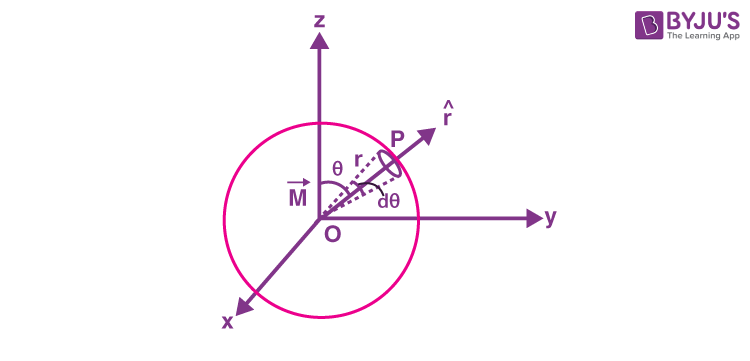2. Three identical bar magnets are riveted together at the centre in the same plane, as shown in Fig. 5.1. This system is placed at rest in a slowly varying magnetic field. It is found that the system of magnets does not show any motion. The north-south poles of one magnet are shown in Fig. 5.1. Determine the poles of the remaining two.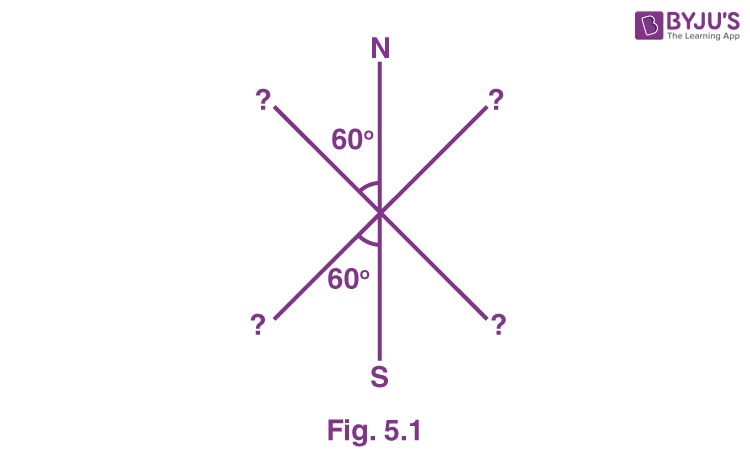Solution: The poles must be symmetric to each other or a magnet.

It is possible only when the poles of the remaining two magnets are as in the given figure.

The north pole of the magnet (1) is equally attracted by the south poles of (2) and (3) magnetic poles placed at equal distances.

Similarly, one pole of any one magnet is attracted by opposite poles of the other two magnets, so the resultant force or moment of each magnet is zero and will not be in motion placed on the table.

3. Suppose we want to verify the analogy between electrostatic and magnetostatic by an explicit experiment. Consider the motion of (i) electric dipole p in an electrostatic field E and (ii) magnetic dipole m in a magnetic field B. Write down a set of conditions on E, B, p, m so that two motions are verified to be identical. (Assume identical initial conditions)

Solution:

$$\begin{array}{l} \textup{ Consider } \theta \textup{ as the angle between } \vec{ m } \textup{ and } \vec{ B } \end{array}$$

Therefore, the torque on the magnetic dipole in a magnetic field B is

$$\begin{array}{l} \tau \vec{ m } \vec{ B } \sin \theta \textup{ …..(i)} \end{array}$$

Likewise, θ is the angle between electric field E and electric dipole moment p, then torque on an electric dipole in E is

$$\begin{array}{l}\tau = \vec{ p } \vec{ E } \sin \theta \textup{ ….(ii)} \end{array}$$

When motion in equations (ii) and (i) of the magnetic and electric dipole are similar, then

$$\begin{array}{l} \tau ‘ = \tau \end{array}$$

$$\begin{array}{l} \vec{ p } \vec{ E } \sin \theta = \vec{ m } \vec{ B } \sin \theta \end{array}$$

$$\begin{array}{l} \vec{ p } \vec{ E } = \vec{ m } \vec{ B } \textup{ …..(ii)} \end{array}$$

It is known that

$$\begin{array}{l} \vec{ E } = \vec{ c } \vec{ B } \textup{ (relation between B and E) ….(iv) } \end{array}$$

The velocity of light is represented by ‘c’.

4. A bar magnet of magnetic moment m and moment of inertia I (about the centre, perpendicular to length) is cut into two equal pieces perpendicular to the length. Let T be the period of oscillations of the original magnet about an axis through the midpoint, perpendicular to the length, in a magnetic field B. What would be the similar period ‘T’ for each piece?

Solution:

The moment of inertia of a bar magnet of mass m, length l about an axis passing through its centre and perpendicular to its length is I=ml212. Let M be the magnetic moment of the magnet, B is the uniform magnetic field in which the magnet is oscillating, and then the time period of oscillation is

$$\begin{array}{l} T = 2 \pi \sqrt{ \frac{ I }{ MB } } \end{array}$$

When a magnet is beaked into two identical pieces, then the moment of inertia of each piece of magnet about an axis normal to length going through its centre is

$$\begin{array}{l} I ‘ = \frac{ m }{ 2 } \frac{ (l / 2) ^ { 2 } }{ 12 } \end{array}$$

$$\begin{array}{l} = \frac{ m l ^ { 2 } }{ 12 } \times \frac{ 1 }{ 8 } = \frac{ I }{ 8 } \end{array}$$

Magnetic dipole moment,

$$\begin{array}{l} M ‘ = \frac{ M }{ 2 } \end{array}$$

The oscillation time period is

$$\begin{array}{l} T ‘ = 2 \pi \sqrt{ \frac{ I’ }{ M’ B } } \end{array}$$

$$\begin{array}{l} = 2 \pi \sqrt{ \frac{ I / 8 }{ ( M / 2) B } } \end{array}$$

$$\begin{array}{l} = \frac{ 2 \pi }{ 2 } \sqrt{ \frac{ I }{ M B } } \end{array}$$

$$\begin{array}{l} = \frac{ T }{ 2 } \end{array}$$

5. Use (i) Ampere’s law for H and (ii) continuity of lines of B to conclude that inside a bar magnet, (a) lines of H run from the N pole to the S pole, while (b) lines of the B must run from the S pole to N pole.

Solution: Let’s take a magnetic field line B through the magnet bar as represented in the diagram. It should be a closed loop.

Take an Amperian loop C outside and inside the magnet NS on the side PQ of the magnet then

$$\begin{array}{l} \int _ { P } ^ { Q } \vec{ H } . \vec{ dl } = \int _ { Q } ^ { P } \frac{ \vec{ B } }{ \mu _ { 0 } } \vec{ dl } \end{array}$$

Here, m0 is the dipole moment, and B is the magnetic field. Since the angle between dl and B changes from 90o, 0, 90o from R to T in the diagram, therefore, cos θ is larger than 1. Thus,

$$\begin{array}{l} \int _ { P } ^ { Q } \vec{ H } . \vec{ dl } = \int _ { Q } ^ { P } \frac{ \vec{ B } }{ \mu _ { 0 } . \vec{ dl } } > 0 \,\,\, ( \textup{positive} ) \end{array}$$

Therefore, the value of B should be changed from the south pole to the north pole in the magnet.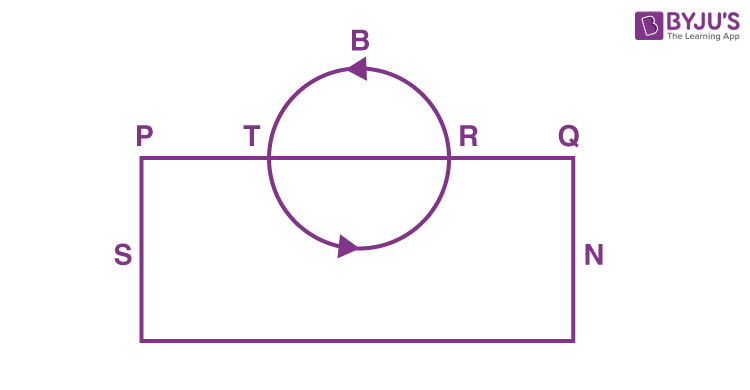1. Verify Ampere’s law for the magnetic field of a point dipole of dipole moment m = mk. Take C as the closed curve running clockwise along (i) the z-axis from z = a > 0 to z = R;

(ii) along the quarter circle of radius R and centre at the origin, in the first quadrant of the x-z plane; (iii) along the x-axis from x = R to x = a, and (iv) along the quarter circle of radius a and centre at the origin in the first quadrant of the x-z plane.

Solution: From point P to Q, all points on the z-axis exist at the axial line of the magnetic dipole of moment M (vector). The magnetic field induction at a point length z from the magnetic dipole moment M (vector) is

$$\begin{array}{l} \left| \vec{ B } \right| = \frac{ \mu _ { 0 } }{ 4 \pi } \frac{ 2 \left| \vec{ M } \right| }{ z ^ { 3 }} \end{array}$$

$$\begin{array}{l} = \frac{ \mu _ { 0 } M }{ 2 \pi z ^ { 3 } } \end{array}$$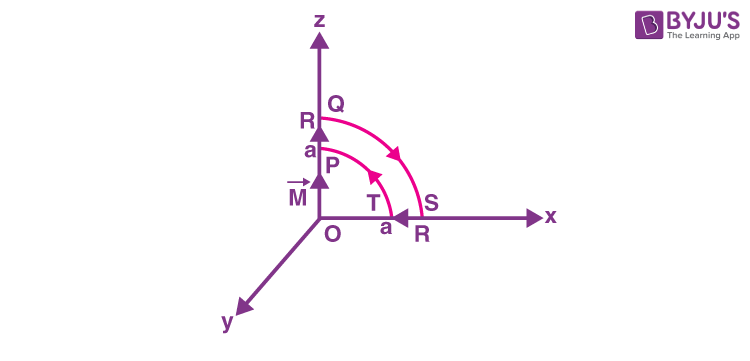(i) Across z-axis from point to Q,

$$\begin{array}{l} \int _ { P } ^ { Q } \vec{ B } . \vec{ dl } = \int _ { P } ^ { Q } B dl \cos 0 ^ { o } \end{array}$$

$$\begin{array}{l} = \int _ { a } ^ { R } B dz \end{array}$$

$$\begin{array}{l} = \int _ { a } ^ { R } \frac{ \mu _ { 0 } }{ 2 \pi } \frac{ M }{ z ^ { 3 } } dz \end{array}$$

$$\begin{array}{l} = \frac{ \mu _ { 0 } M }{ 2 \pi } \left ( – \frac{ 1 }{ 2 } \right ) \left ( \frac{ 1 }{ R ^ { 2 } } – \frac{ 1 }{ a ^ { 2 } } \right ) \end{array}$$

$$\begin{array}{l} = \frac{ \mu _ { 0 } M }{ 4 \pi } \left ( \frac{ 1 }{ a ^ { 2 }} – \frac{ 1 }{ R ^ { 2 } } \right ) \end{array}$$

(ii) Across the quarter circle QS (radius R), A exists on the equatorial line of the magnetic dipole of moment Msinθ.

The magnetic field at A on the circular arc is

$$\begin{array}{l} B = \frac{ \mu _ { 0 } }{ 4 \pi } \frac{ M \sin \theta }{ R ^ { 3 } } \end{array}$$

$$\begin{array}{l} dl = R d \theta \end{array}$$

$$\begin{array}{l} \Rightarrow \int _ { \textup{circular arc QS} } \vec{B . \vec{ dl } } = \int B dl \cos 0 \end{array}$$

$$\begin{array}{l} = \int _ { 0 } ^ { \pi / 2 } \frac{ \mu _ { 0 } }{ 4 \pi } \frac{ M \sin \theta }{ R ^ { 3 } } R d \theta \end{array}$$

$$\begin{array}{l} = \frac{ \mu _ { 0 } }{ 4 \pi } \frac{ M }{ R ^ { 2 } } ( – \cos \theta ) ^ { \pi / 2 } _ { 0 } \end{array}$$

$$\begin{array}{l} = \frac{ \mu _ { 0 } }{ 4 \pi } \frac{ M }{ R ^ { 2 } } \end{array}$$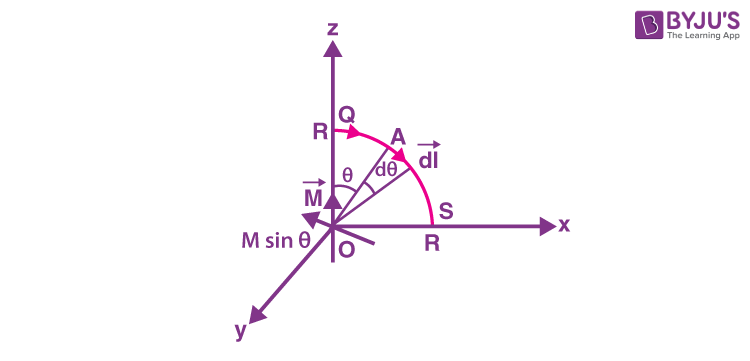(iii) Across the x-axis over the ST path, all points exist on the equatorial line of a magnetic dipole. The magnetic field at an arbitrary point length x from the dipole is

$$\begin{array}{l} \vec{ B } = – \frac{ \mu _ { 0 } }{ 4 \pi } \frac{ \vec{ M } }{ x ^ { 3 } } \end{array}$$

$$\begin{array}{l} \Rightarrow \int _ { S } ^ { T } B . \vec{ dl } = \int _ { R } ^ { a } – \frac{ \mu _ { 0 } \vec{ M } }{ 4 \pi x ^ { 3 } } . \vec{ dl } = 0 \end{array}$$

$$\begin{array}{l} [ \because \textup{ angle between } ( – \vec{ M }) \textup{ and } \vec{ dl } \textup{ is } 90 ^ { o }] \end{array}$$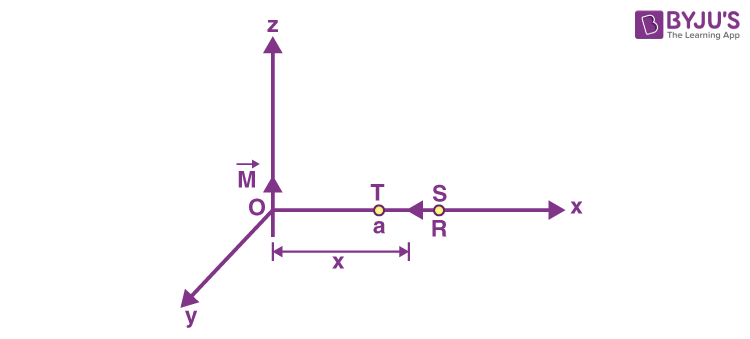(iv) Across the quarter circle TP (radius a), the line integral of vector B along the quarter circle TP is

$$\begin{array}{l} \int _ { \textup{ circular arc TP } } \vec{ B } . \vec{ dl } = \int _ { \pi / 2 } ^ { 0 } \frac{ \mu _ { 0 } }{ 4 \pi } \frac{ M \sin \theta }{ a ^ { 3 } } \, a \, d \theta \end{array}$$

$$\begin{array}{l} = \frac{ \mu _ { 0 } }{ 4 \pi } \left ( \frac{ M }{ a ^ { 2 } } \right ) \int _ { \pi / 2 } ^ { 0 } \sin th \eta d \theta \end{array}$$

$$\begin{array}{l} = \frac{ \mu _ { 0 } }{ 4 \pi } \frac{ M }{ a ^ { 2 } } [ – \cos \theta ] _ { \pi / 2 } ^ { 0 } \end{array}$$

$$\begin{array}{l} = – \frac{ \mu _ { 0 } }{ 4 \pi } \frac{ M }{ a ^ { 2 } } \end{array}$$

$$\begin{array}{l} \Rightarrow \oint _ { P Q S T } \vec{ B } . \vec{ d l } = \int _ { P } ^ { Q } ec B . \vec{ d l } + \int _ { Q } ^ { S } \vec{ B } . \vec{ d l } + \int _ { S } ^ { T } t \vec{ B } . \vec{ d l } + \int _ { T } ^ { P } \vec{ B } . \vec{ d l } \end{array}$$

$$\begin{array}{l} = \frac{ \mu _ { 0 } M }{ 4 \pi } \left ( \frac{ 1 }{ a ^ { 2 } } – \frac{ 1 }{ R ^ { 2 } } \right ) + \frac{ \mu _ { 0 } }{ 4 \pi } \frac{ M }{ R ^ { 2 } } + 0 + \left ( – \frac{ \mu _ { 0 } }{ 4 \pi } \frac{ M }{ a ^ { 2 } } \right ) \end{array}$$

= 0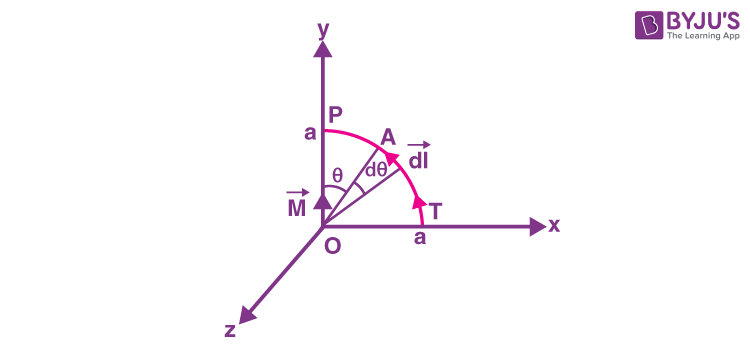2. What are the dimensions of χ, the magnetic susceptibility? Consider an H-atom. Guess an expression for χ, up to a constant, by constructing a quantity of dimensions of χ out of parameters of the atom: e, m, v, R, and µ0. Here, m is the electronic mass, v is electronic velocity, and R is Bohr radius. Estimate the number so obtained and compare it with the value of |χ| ~ 10-5 for many solid materials.

Solution: Since I and H both have the same dimensions and units; thus X has no dimensions.

In this case, X is to be connected with μ0, R, v, m, and e. It is known that the dimensions of

$$\begin{array}{l} \mu _ { 0 } = [ M < h \eta ^ { -1 } ] \end{array}$$

According to Biot-Savart’s law,

$$\begin{array}{l} d B = \frac{ \mu _ { 0 } }{ 4 \pi } \frac{ I dt \sin \theta }{ r ^ { 2 } } \end{array}$$

$$\begin{array}{l} \mu _ { 0 } = \frac{ 4 \pi r ^ { 2 } d B }{ I dl \sin \theta } = \frac{ 4 \pi r ^ { 2 } }{ i dl \sin \theta } \times \frac{ f }{ q v \sin \theta } \, \, \left [ \textup{because } d B = \frac{ F }{ q v \sin \theta } \right ] \end{array}$$

$$\begin{array}{l} \textup{Therefore, the dimensions of } \mu _ { 0 } = \frac{ L ^ { 2 } \times ( M L T ^ { -2 } ) }{ ( q T ^ { -1 } ) ( L ) \times 1 \times ( Q ) ( L T ^ { -1 } ) \times ( 1 ) } \end{array}$$

$$\begin{array}{l} = [ M L Q ^ { -2 } ] \end{array}$$

Here, Q is the charge’s dimension.

$$\begin{array}{l} \textup{Let } X = \mu _ { 0 } \theta ^ { 2 } m ^ { a } v ^ { b } R ^ { c } \textup{ ……(i)} \end{array}$$

Here c, b, and a are the powers of R, m, and v, respectively, such that equation (i) is satisfied.

The dimensional equation of i is

$$\begin{array}{l} [ M ^ { 0 } L ^ { 0 } T ^ { 0 } Q ^ { 0 } ] = [ M L Q ^ { -2 } ] x x [ Q ^ { 2 } ] \times ( L T ^ { -1 } ) ^ { b } \times [ L ] ^ { c } \end{array}$$

$$\begin{array}{l} = [ M ^ { 1 + a } + L ^ { 1 + b + c } T ^ { -b } Q ^ { 0 }] \end{array}$$

After comparing the powers of T, L, and M, we get

$$\begin{array}{l} 0 = 1 + a \Rightarrow a = -1, \end{array}$$
$$\begin{array}{l} 1 + b + c = 0 \end{array}$$
$$\begin{array}{l} 1 + 0 + corc = -1 \end{array}$$

By substituting values in equation (i), we get

$$\begin{array}{l} X = \mu _ { 2 } e ^ { 2 } m ^ { -1 } v _ { 2 } R ^ { -1 } = \frac{ \mu _ { 0 } \theta ^ { 2 } }{ m R } \end{array}$$

In this case,

$$\begin{array}{l} \mu _ { 0 } = 4 \pi \times 10 ^ { -7 } T m A ^ { -1 } \end{array}$$

$$\begin{array}{l} e = 1.6 \times 10 ^ { -19 } C \end{array}$$

$$\begin{array}{l} m = 9.1 \times 10 ^ { -31 } kg \end{array}$$

$$\begin{array}{l} R = 10 ^ { -10 } m \end{array}$$

$$\begin{array}{l} X = \frac{ ( 4 \pi \times 10 ^ { -7 } ) \times ( 1.6 \times 10 ^ { -19 } ) ^ { 2 } }{ 9.1 \times 10 ^ { -31 } \times 10 ^ { -10 } } = 10 ^ { -4 } \end{array}$$

$$\begin{array}{l} \Rightarrow \frac{ x }{ X _ { \textup{given solid} } } = \frac{ 10 ^ { -4 } }{ 10 ^ { -5 } } = 10 \end{array}$$

3. Assume the dipole model for the Earth’s magnetic field B, which is given by Bv = vertical component of the magnetic field, is

$$\begin{array}{l} \frac{ \mu_0}{ 4 \pi } \frac{ 2m cos \theta }{r^3} \end{array}$$
Bn = Horizontal component of the magnetic field is
$$\begin{array}{l}\frac{ \mu _0 }{4\pi} \frac{sin \theta m}{r^3} \end{array}$$
θ = 90o – latitude as measured from the magnetic equator. Find the loci of points for which (i) |B| minimum: (ii) dip angle is zero, and (iii) dip angle is ± 45o.

Solution: (i) We know that the vertical component of the magnetic field of the Earth

$$\begin{array}{l} B _ { v } = \frac{ \mu _ { 0 } }{ 4 \pi } \frac{ M \sin \theta }{ r ^ { 3 } } \end{array}$$

The horizontal component of the magnetic field of the Earth

$$\begin{array}{l} B _ { H } = \frac{ \mu _ { 0 } }{ 4 \pi } \frac{ M \sin \theta }{ r ^ { 3 } } \end{array}$$

$$\begin{array}{l} \Rightarrow B = \sqrt{ B ^ { 2 } _ { V } + B ^ { 2 } _ { H } } = \frac{ \mu _ { 0 } }{ 4 \pi } \frac{ M }{ r ^ { 3 } } \sqrt{ 4 \cos ^ { 2 } \theta + \sin ^ { 2 } \theta } \end{array}$$

$$\begin{array}{l} = \frac{ \mu _ { 0 } }{ 4 \pi } \frac{ M }{ r ^ { 3 } } \sqrt{3 cos ^ { 2 } \theta + 1 } \end{array}$$

From the above equations, we can deduce that B is minimum if cosθ = 0 or θ = π/2, which is so at the magnetic equator. So, B is the lowest at the magnetic equator, which is the loci of points.

(ii) When δ is the angle of dip, then

$$\begin{array}{l} \tan \delta = \frac{ B _ { V } }{ B _ { H } } = \frac{ 2 \cos \theta }{ \sin \theta } = 2 \cot \theta \end{array}$$

The angle of dip δ is zero when cotθ = 0 (θ = π/2). Therefore, the angle of dip is zero on the magnetic equator, which is the loci of points.

(iii) When δ is 45o,

$$\begin{array}{l} \tan 45 ^ { o } = 2 \cot \theta = 2 / tan \theta \, \, or \, \, \tan \theta = 2 \end{array}$$
$$\begin{array}{l} or \, \, \theta = \tan ^ { -1 } ( 2 ) \end{array}$$

is the loci of points.

4. Consider the plane S formed by the dipole axis and the axis of the earth. Let P be a point on the magnetic equator and in S. Let Q be the point of intersection of the geographical and magnetic equators. Obtain the declination and dip angles at P and Q.

Solution: The point of intersection between a geographical meridian and a magnetic meridian is referred to as Q. At this point, the angle of dip at P and Q will be zero, as the magnetic meridian is horizontally aligned. The angle between the axis of rotation of the Earth and the magnetic axis is 113 degrees, and the equators of these respective axes are at 90 degrees with each other. Therefore, the angle between the plane of the magnetic field and the geographical plane, known as declination, will be 113 degrees at both P and Q.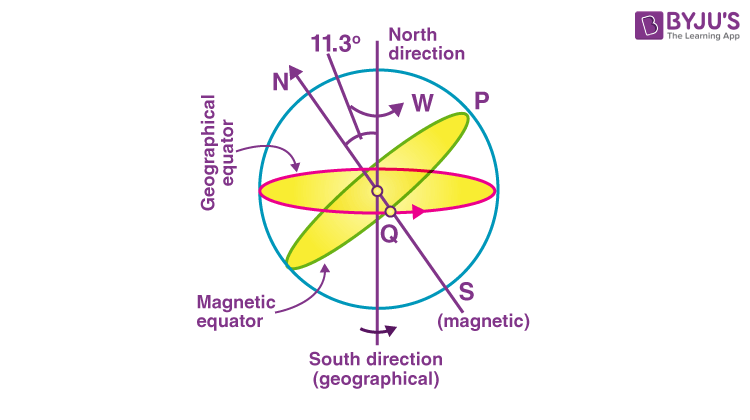5. There are two current-carrying planar coils made each from identical wires of length L. C1 is circular (radius R), and C2 is square (side a). They are so constructed that they have the same frequency of oscillation when they are placed in the same uniform B and carry the same current. Find ‘a’ in terms of R.

Solution: For the coil C1

$$\begin{array}{l} n _ { 1 } = \frac{ L }{ 2 \pi R } \end{array}$$

$$\begin{array}{l} m _ { 1 } = n _ { 1 } i A _ { 1 } = \left ( \frac{ L }{ 2 \pi R } \right ) \,\, ( i ) \,\, ( \pi R ^ { 2 } ) = \frac{ L i R }{ 2 } \end{array}$$

$$\begin{array}{l} I _ { 1 } = \frac{ 1 }{ 2 } M R ^ { 2 } \end{array}$$

I1 is the moment of inertia of coil C1 about an axis through its diameter.

$$\begin{array}{l} \omega _ { 2 } = \sqrt{ \frac{ m _ { 2 } B }{ I _ { 2 } } } \, \, \, as \, \, T _ { 1 } = 2 \pi \sqrt{ \frac{ I _ { 1 } }{ mB } } \end{array}$$

For the coil C2:

$$\begin{array}{l} \textup{Since } n _ { 2 } = \frac{ L }{ 4 a }, m _ { 2 } = n _ { 2 } i A _ { 2 } = \left ( \frac{ L }{ 4 a } \right ) \, \, ( i ) ( a ^ { 2 } ) = \frac{ L i a }{ 4 } \end{array}$$
$$\begin{array}{l} I = \frac{ 1 }{ 12 } M a ^ { 2 } \end{array}$$

I2 is the moment of inertia of coil C2 about an axis through its centre and parallel to one of its sides.

$$\begin{array}{l} \omega _ { 2 } = \sqrt{\frac{ m _ { 2 } B }{ I _ { 2 } } } \end{array}$$

$$\begin{array}{l} \textup{As } \omega _ { 1 } = \omega _ { 2 }, \frac{ m _ { 1 } }{ I _ { 1 } } = \frac{ m _ { 2 } }{ I _ { 2 } } \end{array}$$

$$\begin{array}{l} \textup{or } \frac{ L i R/2 }{ \frac{ 1 }{ 2 } M R ^ { 2 } } = \frac{ L i a/4 }{ \frac{ 1 }{ 12 } M a ^ { 2 } } \textup{ or } \frac{ L i }{ M R } = \frac{ 3 L i }{ M a } \end{array}$$

Thus, a = 3R.

6. Write an essay on ferromagnetism.

Solution: The capability of certain materials to form magnets and be drawn to them is known as ferromagnetism. Ferromagnetism is the fundamental process that allows specific materials, such as iron, to form permanent magnets or be drawn to magnets. Physics recognises several forms of magnetism, with ferromagnetism being the strongest and only type that generates forces powerful enough to be perceived. This type of magnetism is responsible for the ubiquitous magnetic phenomena that we experience in daily life.

When a magnet is close to a ferromagnetic material that isn’t magnetised, the closest opposite poles will cause local magnetisation of the material. The area inside the material that acts like a tiny bar magnet is known as a domain. Individual atoms within domains have their poles aligned, each functioning as a miniature bar magnet. The domains themselves are of small size and randomly positioned in an unmagnetised piece of ferromagnetic material.

The domains can become larger, aligning themselves under the influence of an external magnetic field. This magnetisation can be made permanent through heating and cooling or tapping in the presence of other magnets. Materials that retain magnetisation after being subjected to an external magnetic field and materials that exhibit noticeable attraction to magnets are both considered ferromagnetic.

Ferromagnetism is a result of electrons having a fundamental property that also allows them to possess a dipole moment and carry a charge. Therefore, an electron itself acts as a magnet. The electron’s dipole moment stems from its quantum mechanical spin, which is a more basic property. The electron can only exist in two states, “up” or “down,” due to the quantum mechanical nature of its spin and its relation to the magnetic field. The alignment of tiny magnetic dipoles leads to the combination of their individual magnetic fields, resulting in a measurable macroscopic field.

Materials that have completed electron shells exhibit a zero total dipole moment due to the pairing of electron spins in an “up” and “down” configuration. Ferromagnetism only exists in materials with partially filled electron shells, as only such materials have a net magnetic moment because of the presence of unpaired spins. (Hund’s rules state that the initial electrons in a shell have a tendency to have the same spin, which leads to an increased total dipole moment. )

Ferromagnetism plays a crucial role in various industries and modern technologies, serving as the foundation for devices such as electromagnets, motors, transformers, generators, and magnetic storage tools like hard disks and tape recorders.

## Recommended Videos

#### Magnetism and Matter Class 12 Physics – Full Chapter Revision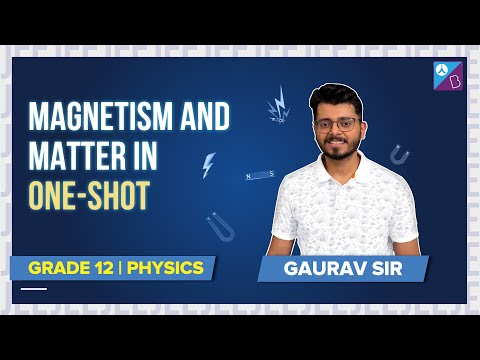#### Magnetism and Matter Class 12 – Top 15 Most Expected JEE Questions#### Magnetism Class 12 JEE Physics Questions, Numericals, and Problems#### Magnetic Properties of Materials Class 12 Physics ConceptsStay tuned to BYJU’S and Fall in Love with Learning!